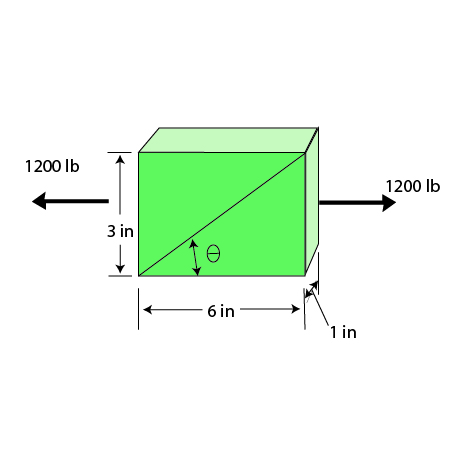# The polyvinyl chloride bar is subjected to an axial force of 1200 lb. If it has the original...

## Question:

The polyvinyl chloride bar is subjected to an axial force of 1200 lb. If it has the original dimensions shown, determine the value of Poisson's ratio if the angle? decreases by ?? = 0.01 after the load is applied. Epvc = 800(103) psi.## Poisson's ratio

In engineering mechanics, the ratio of lateral strain to linear strain is known as Poisson's ratio. here lateral strain means a change in dimension towards y-direction and linear strain means a change in dimension towards X-direction.

Given

Axial force{eq}F = 1200\;{\rm{lb}} {/eq}

{eq}\begin{align*} {x_o} &= 6\;{\rm{in}}\\ {{\rm{y}}_o} &= {\rm{3}}\;{\rm{in}}\\ {{\rm{z}}_o} &= 1\;{\rm{in}}\\ {{\rm{E}}_{pvc}} &= 800 \times {10^3} \end{align*} {/eq}

Angle decreased by{eq}0.01^\circ {/eq}

Here stress in X nd Y direction is Zero.so{eq}{\sigma _x} = {\sigma _y} = 0 {/eq}

Value of Strain is

{eq}\begin{align*} {\varepsilon _x} &= \dfrac{1}{E}\left( {{\sigma _x} - \mu \left( {{\sigma _y} + {\sigma _z}} \right)} \right)\\ &= \dfrac{{{\sigma _x}}}{E}\\ \& \\ \Delta x &= \dfrac{{{\sigma _x}}}{E} \times {x_o}\\ &= \dfrac{{400}}{{800 \times {{10}^3}}} \times 6\\ &= 0.003 \end{align*} {/eq}

{eq}\begin{align*} {\varepsilon _y} &= \dfrac{1}{E}\left( {{\sigma _y} - \mu \left( {{\sigma _x} + {\sigma _z}} \right)} \right)\\ &= \dfrac{{ - \mu {\sigma _x}}}{E}\\ \& \\ \Delta y &= \dfrac{{ - \mu {\sigma _x}}}{E} \times {y_o}\\ &= \dfrac{{ - \mu 400}}{{800 \times {{10}^3}}} \times 3\\ &= - 0.0015\mu \end{align*} {/eq}

At initially , {eq}{\theta _o} = {\tan ^{ - 1}}(3/6) = 26.565^\circ {/eq}

At final

{eq}\begin{align*} \theta ' &= {\theta _0} - \Delta \theta \\ &= 26.565 - 0.01\\ &= 26.555^\circ \end{align*} {/eq}

Hence

{eq}\begin{align*} \tan \left( {\theta '} \right) = \dfrac{{{y_o} + \Delta y}}{{{x_o} + \Delta x}}\\ 0.49978 &= \dfrac{{3 - 0.0015\mu }}{{5.997}}\\ \mu &= 1.8795 \end{align*} {/eq}